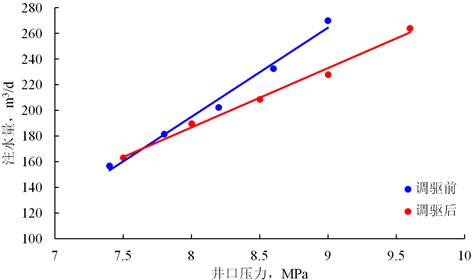﻿ 基于主成分分析法的优势通道识别新方法

# 基于主成分分析法的优势通道识别新方法A New Method to Identify Dominant Channel Based on Principal Component Analysis

Abstract: In order to effectively identify the dominant channel and improve oil recovery in medium and high water cut oilfields, a new method of dominant channel identification based on principal component analysis is proposed. This method can solve the problems of fewer factors and difficult processing in the dominant channel identification method. Thirteen evaluation indicators are selected from the causes of the dominant channel and the dynamic response after the formation of the dominant channel, thus constituting the evaluation index system for identifying the dominant channel. Then, the scattered indicators are integrated into comprehensive indicators by principal component analysis method. Finally, the dominant channel is identified according to the relative size of the comprehensive indicators. This method has been applied in an oilfield block and successfully guided the profile control operation of injection wells. After profile control, the water cut has decreased by 1.20%, and the cumulative oil increase is 11,000 square meters, which provides technical support for further enhancing oil recovery in the oilfield.

1. 引言

2. 评价指标体系的建立Figure 1. Indicator system diagram of dominant channel recognition

3. 主成分分析法的基本原理

4. 实例计算

SZ油田位于渤海辽东湾海域，为湖相三角洲沉积，是典型的高孔、高渗稠油油藏，与一般稠油油藏不同，除了渗透率非均质性突出外，该油田平面流体分布差异也较大。经历了多年的注水开发后，截至2018年底，综合含水已达80%，而采出程度不足30%，优势通道普遍发育，开展优势通道的识别及治理对油田进一步提高采收率意义重大。Table 1. Basic data table for each well group

4.1. 数据无量纲化、同趋势化Table 2. Basic data processing tables for each well group

4.2. 特征值与特征向量计算Table 3. Eigenvalues and characteristic vector tables of evaluation matrix

4.3. 综合评价指标的计算

${\alpha }_{i}=\frac{{\lambda }_{i}}{\underset{i=1}{\overset{p}{\sum }}{\lambda }_{i}}$ (1)

$A=\underset{i=1}{\overset{m}{\sum }}{\alpha }_{i}$ (2)

${F}_{z}={b}_{z1}{Y}_{1}+{b}_{z2}{Y}_{2}+\cdots +{b}_{zp}{Y}_{p}\text{\hspace{0.17em}}\text{\hspace{0.17em}}\text{\hspace{0.17em}}\left(z=1,2,\cdots ,m\right)$ (3)

$F={\alpha }_{1}{F}_{1}+{\alpha }_{2}{F}_{2}+\cdots +{\alpha }_{z}{F}_{z}+\cdots +{\alpha }_{m}{F}_{m}$ (4)Table 4. The channel recognition result tableFigure 2. Contrast diagram of indicating curve before and after driving control in well F6

5. 结论

1) 从优势通道的成因和优势通道形成后的动态响应两个方面出发，筛选构成了识别优势通道的评价指标体系。

2) 利用主成分分析法建立了综合识别优势通道的方法，取所有研究对象的综合评判指标的平均值为评价标准，当井组综合评判指标大于平均值时，认为该井组发育有优势通道。

3) 本文方法在矿场进行了应用，对油田后续进一步提高采收率提供了技术支持。

 曾流芳, 赵国景, 张子海, 等. 疏松砂岩油藏大孔道形成机理及判别方法[J]. 应用基础与工程科学学报, 2002, 10(3): 268-275.

 白振强. 河流-三角洲储层大孔道形成机理研究[J]. 断块油气田, 2007, 14(4): 7-9.

 陈存良, 王振, 牛伟, 等. 基于最小二乘支持向量机的大孔道定量计算方法[J]. 断块油气田, 2015, 22(1): 74-77.

 胡书勇, 张烈辉, 罗建新, 等. 砂岩油藏大孔道的研究——回顾与展望[J]. 特种油气藏, 2006, 13(6): 10-14.

 何长, 李平. 大孔道的表现特征及调剖对策[J]. 石油钻采工艺, 2000, 22(5): 63-66.

 陈存良, 韩晓冬, 赵汉卿, 等. 低渗透油藏井间动态连通性研究[J]. 特种油气藏, 2018, 25(2): 106-110.

 孟凡顺, 黄伏生, 宋德才, 等. 费歇判别法识别大孔道[J]. 中国海洋大学学报: 自然科学版, 2007, 37(1): 121-124.

 史有刚, 曾庆辉, 周晓俊. 大孔道试井理论解释模型[J]. 石油钻采工艺, 2003, 25(3): 48-50.

 窦之林, 曾流芳, 张志海, 等. 大孔道诊断和描述技术研究[J]. 石油勘探与开发, 2001, 28(1): 75-77.

 王森, 冯其红, 宋玉龙, 等. 基于吸水剖面资料的优势通道分类方法——以孤东油田为例[J]. 油气地质与采收率, 2013, 20(5): 99-102.

 王利美, 张国萍, 胡艳霞, 等. 井间示踪剂大孔道识别及剩余油饱和度分布技术[J]. 断块油气田, 2003, 10(4): 72-73.

 刘月田, 孙保利, 于永生. 大孔道模糊识别与定量计算方法[J]. 石油钻采工艺, 2003, 25(5): 54-59.

 何增军, 宋成立, 鲁宁, 等. 模糊综合评判法快速识别低效无效循环井层——以扶余油田西10-2区块为例[J]. 非常规油气, 2016, 3(1): 41-46.

 彭仕宓, 史彦尧, 韩涛, 等. 油田高含水期窜流通道定量描述方法[J]. 石油学报, 2007, 28(5): 79-84.

 黄晓东, 唐晓旭, 刘义刚, 等.新型在线深部调剖技术在海上稠油油田的研究与应用[J]. 非常规油气, 2016, 3(4): 58-64.

 Feng, Q., Wang, S., Wang, S., et al. (2011) Identification of Thief Zones by Dimensionless Pressure Index in Waterfloods. SPE Enhanced Oil Recovery Conference.

 冯其红, 王森, 陈存良, 等. 低渗透裂缝性油藏调剖选井无因次压力指数决策方法[J]. 石油学报, 2013, 34(5): 932-937.

 孟祥海, 刘义刚, 邹剑, 等. 海上稠油油田深部调驱选井决策方法研究[J]. 石油地质与工程, 2017, 31(3): 87-90.

 宋东锋. 东营凹陷南坡湖相碳酸盐岩储层评价方法研究[J]. 非常规油气, 2018, 5(2): 20-27+34.

 王敏, 陈民锋, 刘广为, 等. 主成分分析法确定海上油田水驱效果评价关键指标[J]. 油气地质与采收率, 2015, 22(2): 112-116.

 曲占庆, 张杰, 刘少军, 等. 基于主成分分析法提高有杆抽油系统效率[J]. 断块油气田, 2012, 19(3): 389-392.

Top